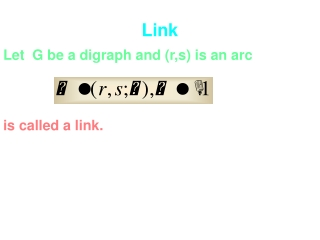DownloadDownload PresentationDownload Presentation- - - - - - - - - - - - - - - - - - - - - - - - - - - E N D - - - - - - - - - - - - - - - - - - - - - - - - - - -
##### Presentation Transcript

1. Link Let G be a digraph and (r,s) is an arc is called a link.

2. positive link is called a positive link r s start end

3. negative link is called a negative link s r start end

4. link chain : a sequece of links is called a link chain eg. path : a sequence of arcs a chain from i to j i j

5. simple chain simple chain : chain with distinct starts for it’s links simple closed path = circuit not simple chain closed chain : i=j, simple closed chain :cycle

6. Singed length Let be a chain length of is called siged length of

7. chain product Let be a chain is called a chain product of A associated If the chain is a cycle, we obtain with a cycle product.

8. V2 V3 Vk V1 Vi Vi+1 i=1,…,k-1 D is called linearly k-partite

9. linearly m-partite cyclically m-partite A digraph is linearly 1-partite it consists of loopless isolated vertices

10. r-cyclic matrix in the superdiagonal block form r-cyclic A square matrix A is r-cyclic if G(A) is cyclically r-partite or equivalently permutation similar

11. block-shift matrix A block-shift matrix is a square matrix in block form with square diagonal blocks s.t. whenever

12. block-shift matrix A square matrix of size greater than one is permutationally similar to a block-shift matrix iff it’s digraph is linearly partite.

13. Union of digraphs digraphs : and is called union of In case is called disjoint union

14. Remark 3.1 The disjoint union of two cyclically m-partite digraphs is cyclically m-partite

15. V2 V3 Vk V1 U1 Uk U2 U3

16. Remark 3.2 A linearly r-partite digraph is cyclically m-partite for any

17. Example for Remark 3.2 A linearly 10-partite digraph is cyclically 4-partite 10=4x2+2

18. Lemma 3.3 The disjoint union of a cyclically m-partite digraph and a linearly s-partite digraph is is a cyclically m-partite digraph.

19. Case 2 s<m

20. Lemma 3.4 The disjoint union of a linearly r-partite digraph and a linearly s-partite digraph is is a linearly t-partite digraph for any positive integer t

21. Lemma 2.7.6 Given G linearly m-partite ( resp. cyclically m-partite) with ordered partition Then every chain from a vertex of to a vertex of is of signed length j-i (resp. j-i (mod m))

22. Remark Let G be a digraph and each of whose cycles has zero signed length then every strongly connected component of G is a single vertex without loop. G is acyclic

23. Remark A is acyclic if and only if

24. Theorem 2.7.7 (i) A digraph is linearly partite if and only if each of cycles has zero signed length.

25. Theorem 2.7.7 (ii) A digraph is cyclically m-partite but not linearly partite if and only if each of its cycles has signed length an integral mutiple of m and it has at least one cycle with nonzero signed length.

26. Exercise Let G be linearly partite and = signed length of the longest chain (i) Prove that if G is linearly s-partite then (ii) G is linearly s-partite for a unique positive integers iff the undirected graph of G is connected . Then

27. Corollary p.1 G is cyclically partite but not linearly partite (1) The cyclic index of G = g.c.d of signed lengths of the cycles of G. (2) G is cyclically m-partite iff m divides the cyclic index of G.

28. Corollary p.2 (3) If the undirected graph of G is connected then up to cyclic rearrangement, the ordered partition of vertex set of G w.r.t. which G is d-cyclically partite is unique where d is the cyclic index of G.

29. Theorem 2.7.10 Let G be a digraph with connected undirected graph and k= g.c.d of signed lengths of cycles of G. Then for vertex x g.c.d of signed lengths of closed chains containing x = k. can not write cycles

30. Theorem 2.7.11 Let A and B are diagonally similar and A, B have the same corresponding cycle products.

31. Theorem p.1 Let Consider (a) A is m-cyclic (b) (c) All cycles of G(A) have signed lengths an integral multiple of m. (d) All circuits of G(A) have lengths an integral multiple of m.

32. Theorem p.2 (e) (f) (g) A and have the same peripheral spectrum.

33. Theorem p.3 Then When G(A) has at least one cycle with nonzero signed length, (a)-(c) are equivalent When A is irreducible, (a)-(d) are equivalent. When A is nonnegative irreducible, then (a)-(g) are equivalent.